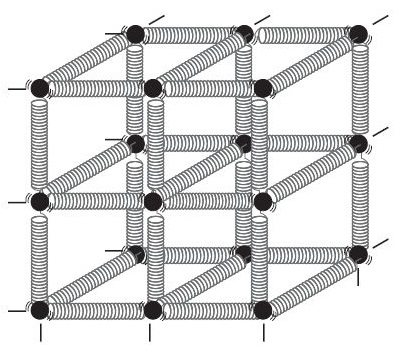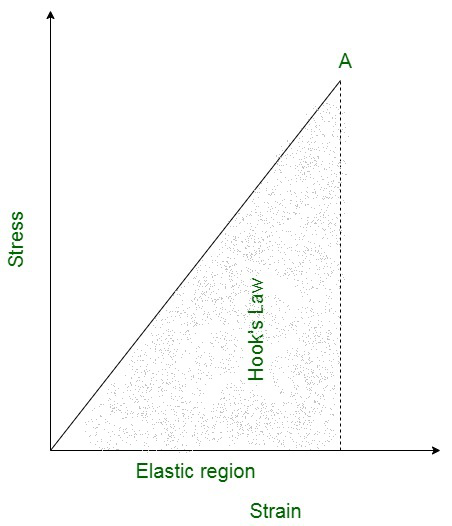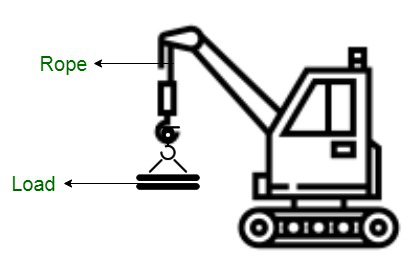# Elastic Behavior of Materials

• Last Updated : 16 Jun, 2021

Solids are made up of atoms based on their atomic elasticity (or molecules). They are surrounded by other atoms of the same kind, which are maintained in equilibrium by interatomic forces. When an external force is applied, these particles are displaced, causing the solid to deform. When the deforming force is removed, interatomic interactions cause the atoms to return to their original state of equilibrium. Elasticity is an idealization since no substance is perfectly elastic. For example, if you use a hair tie to maintain yourself, you may have seen that its size deforms over time. It may snap after a certain point. This is because the hair tie loses its elastic nature over time.

The solids are accustomed to maintaining a physical shape by the virtue of which each molecule contained in it finds itself in a position of stable equilibrium, that is, there are balanced forces acting on the body from every pair of opposite directions. A rigid body is a solid object in which deformation is negligible, assumed to be zero for all practical purposes. These bodies when subjected to an external force restore their original shape after the removal of this force. The body resists the changes, until a limiting external force limit, called the elastic limit.

### Elasticity

Any rigid object in the universe undergoes changes in its physical orientation and structure upon external force application. A rigid body either changes its length, volume or shape when an outward force is applied to it. The body retains its original shape and size upon removal of external acting forces. Therefore, elasticity is such a property of a body by the virtue of which anybody tends to regain its original shape (or) size when external forces are removed. It exhibits an opposition to change. Example: a rubber band.

### Elastic behavior of solids

In the cases, where any solid body is subjected to an external force, the body is deformed and the atoms or molecules forming them are displaced from their original positions, thus being disturbed from the state of equilibrium.

The displacement causes the modification of fixed points, thus, causing a change in the interatomic as well as intra-molecular distances. Therefore, deformation can be termed as the change in the structure of any object due to the influence of a force acting upon it. The force that causes the shift in the displacement of these particles is known as the deforming force

Since we know that every force has an equal and opposite force acting. The deforming force is opposed by the restoring force, acting in the opposite direction. This force pushes the body to regain its original position upon removal of the deforming force.

The spring ball system contains balls denoting atoms and springs representing the forces acting between them.Spring-ball model for the illustration of elastic behavior of solids

### Hooke’s Law

Hooke’s law states that within the elastic limit, stress developed is directly proportional to the strain produced in a body. This implies that if the strain on anybody increases the stress will increase and vice-versa.Hooke’s law is applicable to all bodies exhibiting elastic properties. Mathematically, Hooke’s Law is defined as,

Stress ∝ Strain

Let us assume a constant of

ortionality k.

Therefore,

Stress = k × Strain

Here, the balancing factor k is known as the modulus of elasticity

### Elastic Modulus

Since we know that an elastic material is deformed due to an external force, it maintains an internal resistance to oppose the deformation and restores it to its original state if the external force is no longer applied. There are different types of elastic moduli as:

1. Young’s Modulus: Young’s modulus is the property of an object by the virtue of it withstands changes in its length, in the cases where lengthwise tension or forces, in the form of compression is applied. Young’s modulus is equivalent to the longitudinal stress divided by the strain of the object. It is denoted by Y.
2. Shear Modulus: Also known as Modulus of Rigidity, the shear modulus is defined as the ratio of shearing stress to shearing strain. It is denoted by ‘G’.
3. Bulk Modulus: Bulk modulus is the ratio of hydraulic stress to the corresponding hydraulic strain. Denoted by ‘B’

### Application of Elastic Behavior of Solids

When you stretch a slingshot, it deforms. When you remove the force, it returns to its former shape. But suppose you take a thin steel rod and try to bend it. You bend it slightly and then cease exerting force. Is the rod able to revert to its previous shape? It doesn’t, it doesn’t. This discrepancy in material behavior is due to its elastic and plastic properties

The slingshot’s rubber strip is extremely pliable. Elasticity is a body’s capacity to resist irreversible alteration when subjected to stress. When the tension is removed, the body returns to its former shape and size. Different materials exhibit varying degrees of elasticity. The study of a material’s elastic behavior is very important. Almost every engineering design necessitates an understanding of material elastic behavior. In the building of different constructions such as bridges, columns, pillars, and beams, among others. It is critical to understand the strength of the materials used in building. The elastic behavior of materials is considered and learned mostly in three main cases:

1. Thickness of the Steel Rope used in Cranes.
2. Design of the bridges,
3. Maximum Height of the mountains.

### Thickness of the Steel Rope used in Cranes.

Cranes used to lift loads use ropes that are designed so that the stress due to the maximum load does not exceed the breaking stress. It is also found that a collection of thinner wire strands when compacted together make the rope stronger than a solid rope of the same cross-section. That is why crane ropes are made of several strands instead of one.We can find that how thick rope is required for a crane; The load should not deform the rope permanently. Therefore, the extension should not exceed the elastic limit. Mild steel has a yield strength (Sy) of about 300 × 106 Nm-2.  Thus, the area of cross-section (A) of the rope should at least be:

Elastic limit, Sy = Load to be lifted / Cross-sectional Area = W / A

The load to be lifted by the crane is equal to the weight (W) that is to lift which must be equal to the product of the mass (m) of the weight lifted and gravitational acce

tion (g) that is, mg.

And since, the rope is circular-shaped of radius (r) so, its area of cross-section (A) is πr2.

Therefore,

Sy = W / A = mg / πr

Now, A should be greater than or equals to W / Sy or mg / Sy

i.e.

A ≥ (104 kg × 10 ms2) / (300 × 106 Nm-2)

= 3.3 × 10-4 m2

This corresponds to the radius of about 1 cm for a rope of circular cross-section. Generally, a large margin of safety (of about a factor of ten in the load) is provided. Thus, a thicker rope of radius about 3 cm is recommended.

### Design of the Bridges

A bridge has to be designed in such a way that it should have the capacity to withstand the load of the flowing traffic, the force of winds, and even its own weight.

[Image_Link]https://media.geeksforgeeks.org/wp-content/uploads/20210524

The elastic behavior or in other words the bending of beams is a major concern over the stability of the buildings or bridges. The beam should not bend too much or break, A beam loaded at the Centre and supported near its ends. A bar of length l, breadth b, and depth d, when loaded at the center by a load W, will have depression:

δ = WL3 / 3YIG

where W is the load, L is the length, Y is the Young’s Modulus and IG is the geometrical moment of inertia.

### Maximum Height of the Mountains

Let us assume, the height of the mountains at the bottom is h, the force per unit area due to the weight of the mountain is hρg where ρ is the density of the material of the mountain and g is the acceleration due to gravity.

The material at the bottom experiences this force in the vertical direction, and the sides of the mountain are free. Now the elastic limit for a typical rock is 30 × 107 Nm-2 and put ρ = 3 × 103 kg m-3 therefore, this implies:

hρg = 30 × 107 Nm-2

Rearrange the above expression for h.

Hence, the maximum height of the mountains should be,

h = 30 × 107 Nm-2 / ρ × g

= 30 × 107 Nm-2 / 3 × 103 g m-3 × 10 ms-2

= 10000 m or 10 km

This is why the height of the mountains cannot be higher than 10 km.

### Sample Problems

Problem 1: Give a reason why steel used for the construction of bridges?

Solution:

Steel has the strongest and most favorable strength attributes of any bridging material, making it appropriate for the most daring bridges with the greatest spans. Normal construction steel has compressive and tensile strengths of 370 N/sq mm, which is approximately ten times the compressive strength of medium concrete and one hundred times its tensile strength.

Steel has a unique property called ductility, which allows it to bend significantly before breaking because it begins to give over a specific stress threshold.

Problem 2: Determine the force constant when the interatomic spacing in a steel cable is 2.5 Å and the value of its young’s modulus is 20 × 1010 N/m2.

Solution:

The formula to calculate the force constant is,

K = Y × r0

where Y is the young’s modulus and r0 is the interatomic spacing.

Lets substitute the given values in the above expression as,

K = 20 × 1010 N/m2 × 2.5 Å × (10-10 m / 1 Å)

= 6 × 10−9 N/Å

Problem 3: The stretching of a coil spring is determined by which modulus type and why?

Solution:

Upon stretching a coil spring, a change in its shape is noticed. The length as well as the volume remain unmodified, therefore, it can be determined by evaluation through shear modulus.

Problem 4: Which material shows better elasticity, steel or rubber?

Solution:

Steel is more elastic than rubber because it returns to

original position faster than rubber.

Problem 5: How the dip in the middle of the beam is related to its Young’s modulus while, constructing a beam for use in supporting a load.

Solution:

The relation for the determination of the dip in the middle of the beam is,

δ = WL3 / 3YIG

where W is the load, L is the length, Y is the Young’s Modulus and IG is the geometrical moment of inertia.

However, here if the load, length and geometrical moment of inertia of the beam remains constant then,

δ ∝ 1 / Y

Hence, the dip in the middle of the beam is inversely proportional to its Young’s modulus.

My Personal Notes arrow_drop_up